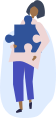Chemistry Homework Help

Get step-by-step solutions from expert tutors.

## How it works?Type, take a picture, or paste your question with all necessary details to ensure high-quality responses.### Get an answer in less than 24hrs

Once our tutors receive the question, they'll begin. You'll get an email once the solution is ready.### Get Notified

We will send you email and text notification to let you know when your question is answered.

### Browse Homework Solutions

244 solutions

Calorimetry

#### Q. The heat of combustion for a sample of coal is 23.0 kJ/g. What quantity of coal (in grams) must be burned to heat 500.0 g of water from 20.0°C to 95.0°C?

Solved • Feb 11, 2020

Calorimetry

#### Q. Consider an aqueous reaction of equal volumes of 2.0 M HCl and 2.0 M NaOH, with a final volume of 100.0 mL and a density of 1.03 g/ml. What will the change in heat (qrxn) be for this reaction given a positive temperature change of 2.5 degrees Celsius? Assume a value of 4.18 J g-1 C-1for Csoln

Solved • Feb 4, 2020

Calorimetry

#### Q. Ice cubes at 0°C with a total mass of 435 g are put in a microwave oven and heated with 750. W (750. J/s) of energy for 6.00 minutes. What is the final temperature of the water from the melted ice? Assume all of the microwave energy is absorbed by the ice/water and no heat loss by the ice/water. The enthalpy of fusion for ice is 6.02 kJ/mol and the heat capacity for water is 4.18 J/g･°C.

Solved • Feb 2, 2020

Calorimetry

#### Q. Which conditions would result in an exothermic ΔHsolution? a.ΔHsolvent>> ΔHsolute d.ΔHsolute< ΔHhydration . b.ΔHsolute> ΔHhydration . e.Not enough information to determine. c.ΔHsolute≈ ΔHhydration

Solved • Jan 26, 2020

Calorimetry

#### Q. Determine ΔHsolute for KBr if the ΔHsolution of KBr is +19.9 kJ/mol and the ΔHhydration of KBr is −670 kJ/mol. a.+650 kJ/mol . d.−710 kJ/mol . b.−650 kJ/mol . e.−690 kJ/mol . c.+690 kJ/mol

Solved • Jan 26, 2020

Calorimetry

#### Q. he salt barium bromide is soluble in water. When 8.51 g BaBr2 is dissolved in 103.00 g water, the temperature of the solution increases from 25.00 °C to 26.50 °C. Based on this observation, calculate the dissolution enthalpy, ΔdissH, of BaBr2. Assume that the specific heat capacity of the solution is 4.184 J g-1 °C-1 and that the energy transfer to the calorimeter is negligible. ΔdissH =__?____ kJ/mol ****I got 24.10 but it is saying its wrong

Solved • Jan 24, 2020

Calorimetry

#### Q. The addition of hydrochloric acid to a silver nitrate solution precipitates silver chloride, When you combine 50.0ml of 0.100M silver nitrate with 50.0 ml of hydrochloric acid in a coffee cup calorimeter, the temperature changes from 23.40 degrees celsius to 24.21 degrees celsius, Write a balanced chemical equation and calculate delta Hrxn, Use 1.00g/ml as the density of the solution and Cs = 4.18J/g*degrees celsius as the specific heat capacity.

Solved • Jan 21, 2020